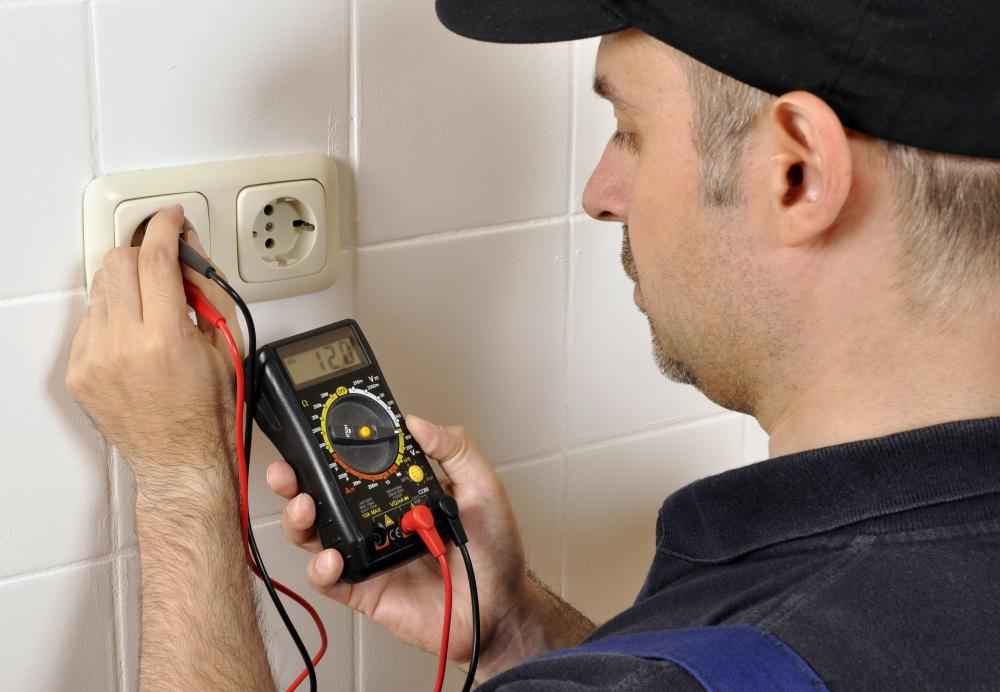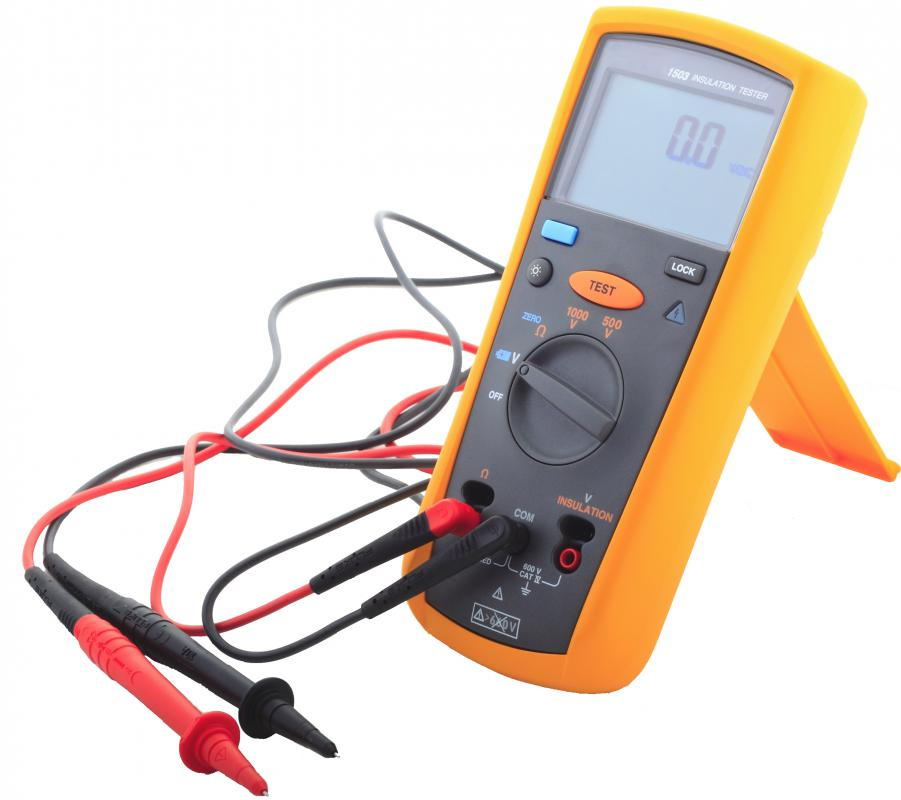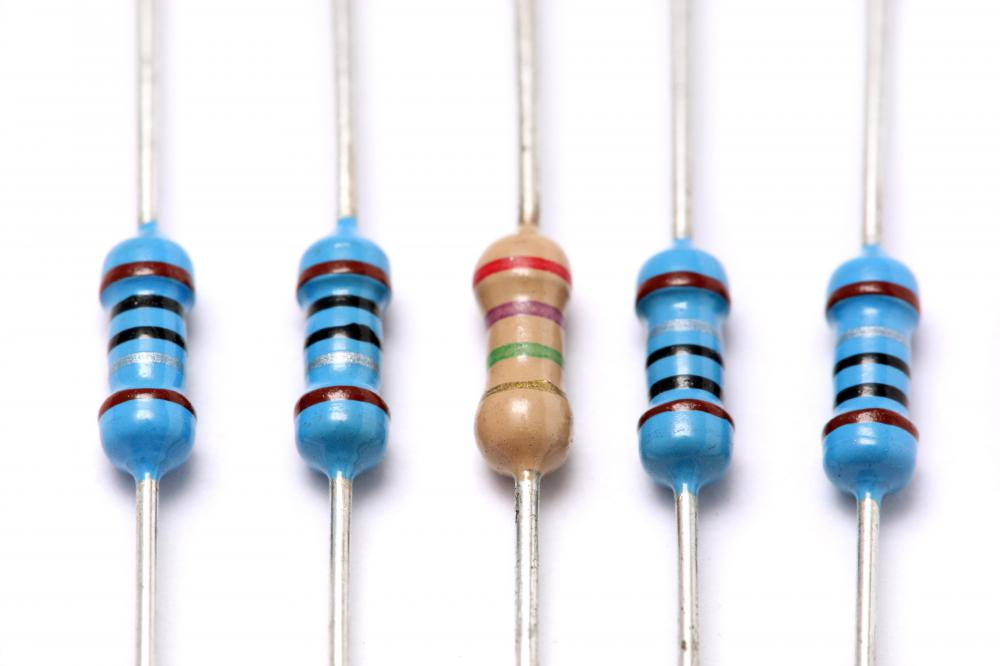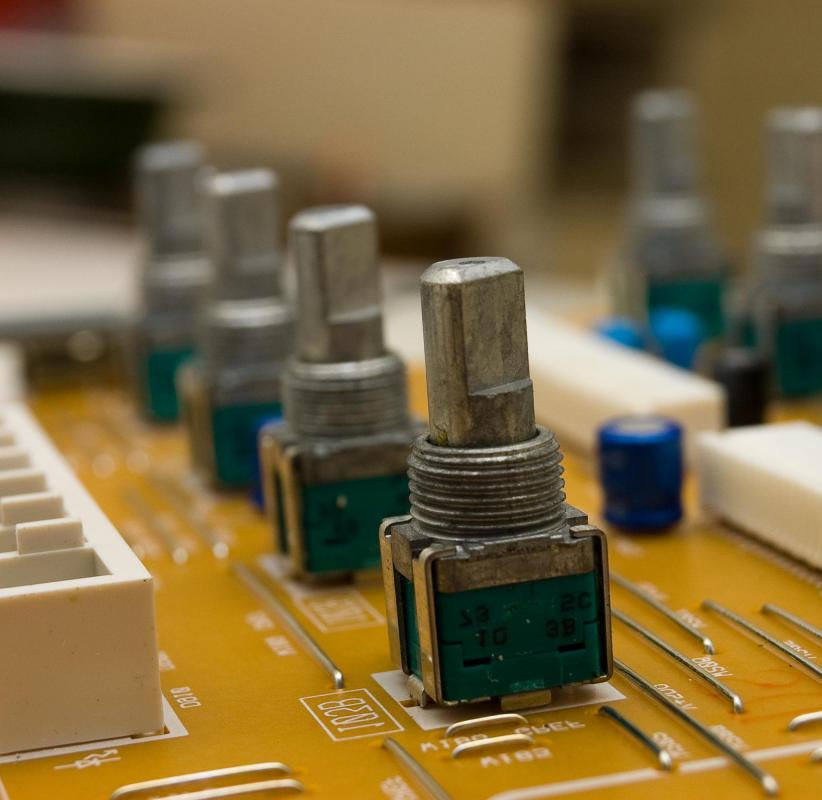# How do Ohmmeters Work?

Donna Rengi
Donna RengiAn ohmmeter can be used to check an electrical connection's continuity.

Ohmmeters are electrical devices used for measuring the resistance of a given conductor. This measuring instrument works on the basis of Ohm’s Law, which applies to electrical circuits. According to this law, the current (I) that flows between two points in a conductor is directly proportional to the voltage (V) or potential difference across the two points. It is also inversely proportional to the resistance (R) between them. Therefore, mathematically, V = IR.A digital multimeter is a tool that can be used as an ohmmeter.

In order to measure the resistance of a given conductor, the red and black leads of an ohmmeter are connected to the positive and negative terminals of the conductor, respectively. The resistance of the wire or circuit is indicated by a needle sliding on the device's scale. These meters measure the resistance in Ohms, denoted by the Greek capital letter omega or Ω.Resistors are electrical devices that manage the flow of current through a circuit.

An ohmmeter should never be connected to a voltage source, as it can damage the equipment. This is because the device already has a source that supplies voltage for measuring the resistance of a given conductor. The resistance is measured according to the voltage drop across the terminals of the conductor. In an analog meter, the far left-hand side of the scale indicates infinite resistance and the far right-hand side denotes zero resistance.Variable resistors are attached to a battery and moving meter in an ohmmeter.

A simple analog device consists of a battery, which is the voltage source, connected to a moving meter. A variable resistor is also connected in series to this combination so that the needle exactly shows a full scale deflection and does not slide beyond the zero resistance mark. This resistor also limits the current and corrects for the decrease in voltage caused by the aging of the battery. Analog ohmmeters must be calibrated before use, whereas digital ones can usually self-calibrate.

In order to calibrate an analog meter, both the leads must be held together. The adjustment knob helps in setting the variable resistor. It must be manually turned so that the needle indicates zero resistance; in other words, the needle now rests at the far right-hand side. This step is known as "zeroing" the meter, and it should be repeated every time before the resistance of any wire or circuit is measured. In the case of a digital device, holding the leads together will indicate 0 Ω, which is sufficient for its calibration.

In addition to resistance measurement, ohmmeters can be used to check the continuity of an electrical connection. For instance, if the needle rests on infinite resistance on far left of the scale, it indicates no continuity in the circuit. This implies that there is an open point in the circuit. On the other hand, if the measured resistance value is zero or far less than the expected value, it implies a short in the circuit.

## You might also Like## Discussion Comments

anon316802

Ohmeters actually can be connected to voltage sources. Internally, the impedance between the test leads is very large, and almost no current flow occurs. Most ohmeters even have a setting to measure the voltage of the source. (See the little V on the dial of the stock image above).

anon304044

How is an ohmmeter is constructed and how does it work?

Forgot password?
###### Register:
•An ohmmeter can be used to check an electrical connection's continuity.
•A digital multimeter is a tool that can be used as an ohmmeter.
•Resistors are electrical devices that manage the flow of current through a circuit.
•Variable resistors are attached to a battery and moving meter in an ohmmeter.